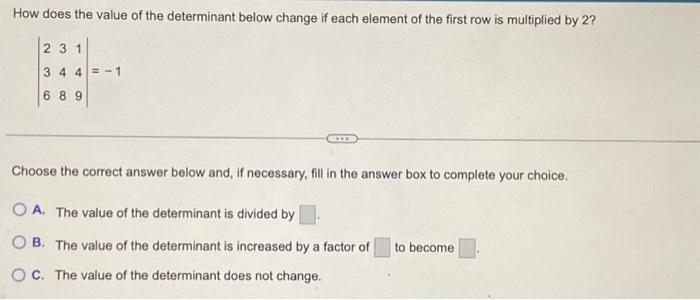Home / Expert Answers / Algebra / how-does-the-value-of-the-determinant-below-change-if-each-element-of-the-first-row-is-multiplied-by-pa546

# (Solved): How does the value of the determinant below change if each element of the first row is multiplied by ...

How does the value of the determinant below change if each element of the first row is multiplied by 2? 23 1 344-1 689 *** Choose the correct answer below and, if necessary, fill in the answer box to complete your choice. OA. The value of the determinant is divided by OB. The value of the determinant is increased by a factor of to become OC. The value of the determinant does not change.How does the value of the determinant below change if each element of the first row is multiplied by 2 ? Choose the correct answer below and, if necessary, fill in the answer box to complete your choice. A. The value of the determinant is divided by B. The value of the determinant is increased by a factor of to become C. The value of the determinant does not change.

We have an Answer from Expert